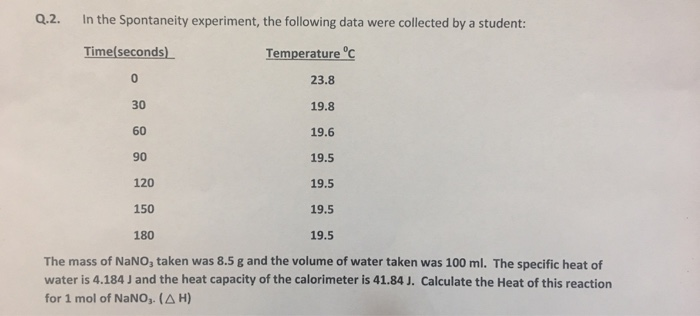# Question & Answer: In the Spontaneity experiment, the following data were collected by a student: The mass of…..In the Spontaneity experiment, the following data were collected by a student: The mass of NaNO_3 taken was 8.5 g and the volume of water taken was 100 ml. The specific heat of water is 4.184 J and the heat capacity of the calorimeter is 41.84 J. Calculate the Heat of this reaction for 1 mol of NaNO_3 (Delta H)

The final temperature of the water is obtained graphically.

Plot of temperature vs time

Don't use plagiarized sources. Get Your Custom Essay on
Question & Answer: In the Spontaneity experiment, the following data were collected by a student: The mass of…..
GET AN ESSAY WRITTEN FOR YOU FROM AS LOW AS \$13/PAGE

The intersection of the Series 2 line with the temperature axis (by extrapolation) gives the final temperature of the system.

Put x = 0 in the linear regression equation (Series 2) and obtain

y = -0.0017*(0) + 19.747

===> y = 19.747 ≈ 19.7

The final temperature of the solution is 19.7⁰C.

Assume the density of water is 1 g/mL so that the mass of water taken = (100 mL)*(1 g/mL) = 100 g.

The mass of the solution, ms = (8.5 + 100.0) g = 108.5 g; the specific heat of the solution is Cs = 4.184 J/g.⁰C and the change in temperature = tf – ti = Δt = (19.7 – 23.8)⁰C = -4.1⁰C.

The energy transferred from the solution is qs = m2*Cs*Δt = (108.5 g)*(4.184 J/g.⁰C)*(-4.1⁰C) = -1861.2524 J.

The energy transferred from the calorimeter is qcal = Ccal*Δt where Ccal = heat capacity of the calorimeter = (41.84 J/⁰C)*(-4.1⁰C) = -171.544 J.

The energy change for the reaction is qrxn = -(qs + qcal) = -[(-1861.2524 J) + (-171.544 J)] = -2032.7964 J.

The molar mass of NaNO3 = (1*23 + 1*14 + 3*16) g/mol = 85 g/mol; the moles of NaNO3 corresponding to 8.5 g = (8.5 g)/(85 g/mol) = 0.1 mol.

The heat of reaction for 1 mole of NaNO3 is given as (2032.7964 J)*(1.0 mol/0.1 mol) = 20327.964 J = (20327.964 J)*(1 kJ/1000 J) = 20.327964 kJ ≈ 20.33 kJ (ans).